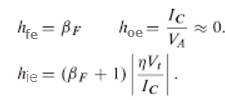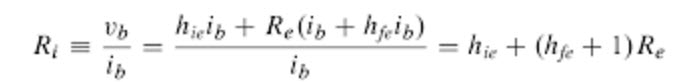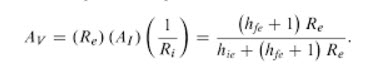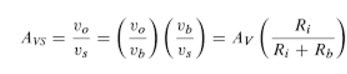# Common Collector Amplifier Circuit and Its Applications

The amplifier is an electronic circuit that is used for amplifying a voltage or current signal. The input for the transistor will be a voltage or current and the output will be an amplified form of that input signal. An amplifier circuit is generally designed with one or more transistors is called a transistor amplifier. The transistor (BJT, FET) is a major component in an amplifier system. In this article, we will discuss the common-collector amplifier circuit.

The Transistor amplifiers are most commonly using in our day to day life applications like an audio amplifier, Radio Frequency, audio tuners, Optical fiber communication, etc.

## Common Collector/ Emitter Follower Transistor Amplifier Basics

As we discussed in our previous article, there are three transistor configurations that are used commonly for signal amplification i.e. common base (CB), common collector (CC) and common emitter (CE).

Good transistor amplifiers essentially have the following parameters high gain, high input impedance, high bandwidth, high slew rate, high linearity, high efficiency, high stability, etc.

In the Common Collector transistor configuration, we use the collector terminal as common for both input and output signals. This configuration is also known as emitter follower configuration because the emitter voltage follows the base voltage. The emitter follower configuration is mostly used as a voltage buffer. These configurations are widely used in impedance matching applications because of their high input impedance.

Common collector amplifiers have the following circuit configurations.

• The input signal enters the transistor at the base terminal
• The input signal exits the transistor at the emitter terminal
• The collector is connected to a constant voltage, i.e. ground, sometimes with an intervening resistor

A simple common-collector amplifier circuit is shown in the figure below. The collector resistor Rc is unnecessary in many applications. In order to work transistor as an amplifier, it should be in the active region of its configuration.

For that we set the quiescent point need to be set with the circuitry external to the transistor, the values of resistors Rc and Rb, and the DC voltage sources, Vcc and Vbb, have chosen accordingly.

Once the circuit quiescent conditions have been calculated and it has been determined that the BJT is in the forward- active region of operation, the h-parameters are calculated below to form the small-signal model of the transistor.### Common Collector Transistor Amplifier Characteristics

The load resistor in the common collector amplifier being placed in series with the emitter circuit receives both the base current and collector currents.

Since the emitter of a transistor is the sum of base and collector currents, since base and collector currents always add together to form the emitter current, it would be reasonable to assume that this amplifier will have a very large current gain.

The common-collector amplifier has quite large current gain, larger than any other transistor amplifier configuration. The characteristics of cc amplifier as mentioned below.

 Parameter Characteristics Voltage gain Zero Current gain High Power gain Medium Input or output phase relationship Zero degree Input resistance High Output resistance Low

The small-signal circuit performance can now be calculated. Total circuit performance is the sum of quiescent and small-signal performance. The AC model circuit is shown below.

#### Current gain

The current gain is defined as the ratio of the load current to the input current.

Ai= il/ib= -ie/ib

From the h-parameter circuit, it can be determined that the emitter and base currents are related through the dependent current source by the constant hfe+1. The current gain is dependent only on the BJT characteristics and independent of any other circuit element values. Its value is given by

Ai= hfe+1

#### Input Resistance

The input resistance is given byThis result is identical to that for a common emitter amplifier with an emitter resistor. The input resistance to a common collector amplifier is large for typical values of the load resistance Re.

#### Voltage Gain

The voltage gain is the ratio of output voltage to input voltage. If the input voltage is again taken to be the voltage at the input to the transistor, Vb.

Av= Vo/Vb

Av= (vo/il)(il/ib)(ib/vb)

Replacing each term with its equivalent expression

Av= (Re)(Ai)(1/Ri)The above equation is somewhat less than unity. The approximation equation of voltage gain is given by

The overall voltage gain can be defined as

Avs= Vo/Vs

This ratio can be directly derived from the voltage gain Av, and a voltage division between the source resistance Rs and the amplifier input resistance RiAfter substitutions of appropriate equations, the overall voltage gain is given by

Avs= 1- (hie+Rb)/(Ri+Rb)

#### Output Resistance

The output resistance is defined as the Thevenin resistance at the output of the amplifier looking back into the amplifier. The circuit is shown below, the AC equivalent circuit to calculate the output resistance.

If a voltage v is applied to the output terminals, the base current is found to be

ib= -v/(Rb+hie)

The total current flowing into the BJT is given by

i= -ib-hfe.ib

the output resistance is calculated as

Ro= v/i = (Rb+hie)/(hfe+1)

The output resistance for a common collector transistor amplifier is typically small.

#### Applications

• This amplifier is used as an impedance matching circuit.
• It is used as a switching circuit.
• The high current gain combined with near-unity voltage gain makes this circuit a great voltage buffer
• It is also used for circuit isolation.